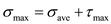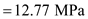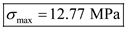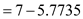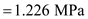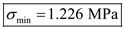# For the state of plane stress shown, determine (a) the value ofτxy for which the in-plane shearing stress parallel to the well] is zero, (b) it the corresponding principal stresses.

Question-AnswerCategory: Strength of MaterialsFor the state of plane stress shown, determine (a) the value ofτxy for which the in-plane shearing stress parallel to the well] is zero, (b) it the corresponding principal stresses.

For the state of plane stress shown, determine (a) the value ofτxy for which the in-plane shearing stress parallel to the well] is zero, (b) it the corresponding principal stresses.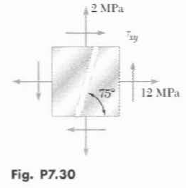Step No: 1Step No: 2

Tensile stress in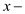direction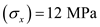Tensile stress indirection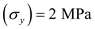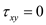Step No: 3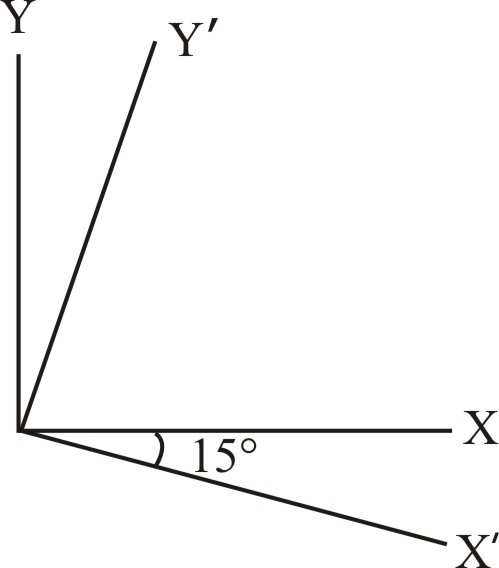Step No: 4

(a) Plane stresses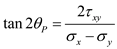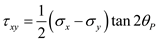Step No: 5

Shear maximum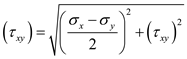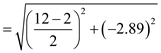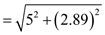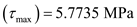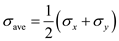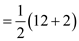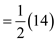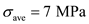Step No: 6

(b) Principal stresses